# procest

Estimate process model using time-domain or frequency-domain data

## Syntax

``sys = procest(tt,type)``
``sys = procest(data,type)``
``sys = procest(___,Name,Value)``
``sys = procest(tt,init_sys)``
``sys = procest(data,init_sys)``
``sys = procest(___,opt)``
``[sys,offset] = procest(___)``
``[sys,offset,ic] = procest(___)``

## Description

### Estimate Process Model

example

````sys = procest(tt,type)` estimates the process model `sys` using all the input and output signals in the timetable `tt`. `type` defines the structure of `sys`. You can use this syntax for SISO and MISO systems. The function assumes that the last variable in the timetable is the single output signal.A simple SISO process model has a gain, a time constant, and a delay:$sys=\frac{{K}_{p}}{1+{T}_{p1}s}{e}^{-{T}_{d}s}.$Kp is a proportional gain. Tp1 is the time constant of the real pole, and Td is the transport delay (dead time). More complex process models can include zeroes, additional time constants, complex poles, and integration. For more information on process models, see `idproc`.You cannot use `procest` to estimate time-series models, which are models that contain no inputs. Use `ar`, `arx`, or `armax` for time-series models instead.You cannot reliably estimate accurate process models from matrix-based data as you can with other model types. Process models are always continuous, and, because numeric matrices contain no sample time information, estimating continuous models from matrix-based data is generally not recommended. For information on converting matrices to timetables, see ```
````sys = procest(data,type)` uses the time-domain or frequency-domain data in `data`. Use this syntax especially when you want to estimate a process model using frequency-domain or frequency response data, or when you want to take advantage of the additional information, such as intersample behavior, data sample time, or experiment labeling, that data objects provide.```

example

````sys = procest(___,Name,Value)` incorporates additional options specified by one or more name-value arguments. For example, `sys = procest(tt,P1D,'InputDelay',2)` specifies an input delay of 2. You can use this syntax with any of the previous input-argument combinations```

### Configure Initial Parameters

example

````sys = procest(tt,init_sys)` uses the process model `init_sys` to configure the initial parameterization for estimation using the timetable `tt`.```

example

````sys = procest(data,init_sys)` uses the uses the data object `data` for estimation.```

example

````sys = procest(___,opt)` specifies additional model estimation options. Use `opt` with any of the input argument combinations in the previous syntaxes.```

### Return Estimated Offset and Initial Conditions

example

````[sys,offset] = procest(___)` returns the estimated value of the offset in input signal. `procest` automatically estimates the input offset when the model contains an integrator or when you set the `InputOffset` estimation option to `'estimate'` using `procestOptions`. ```

example

````[sys,offset,ic] = procest(___)` returns the estimated initial conditions as an `initialCondition` object. Use this syntax if you plan to simulate or predict the model response using the same estimation input data and then compare the response with the same estimation output data. Incorporating the initial conditions yields a better match during the first part of the simulation. ```

## Examples

collapse all

Estimate a process model and compare its response with the measured output.

Load the input/output data, which is stored in the timetable `tt1`.

`load sdata1 tt1`

Estimate a first-order process model `sys` that contains one pole and no zeroes or delays. This model structure has type `P1`.

`sys = procest(tt1,'P1');`

Compare the simulated model response with the measured output.

`compare(tt1,sys)`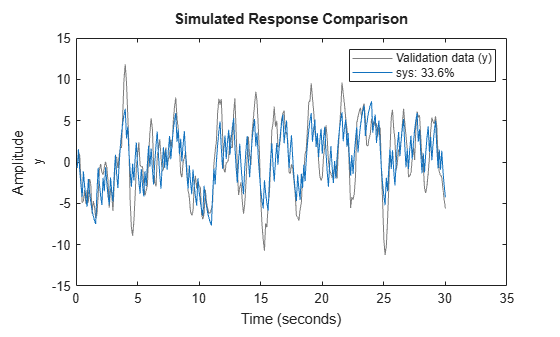The fit percentage for the model is low. Add a delay to the model and compare the simulated and measured outputs.

```sys = procest(tt1,'P1D'); compare(tt1,sys)```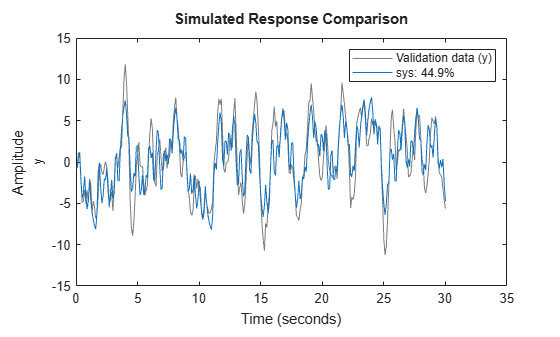The fit percentage has improved, but is still below 50%. The plot shows that the model output peaks do not attain the height of the measured output peaks, which indicates that the model needs to include more dynamics.

Create a second-order process model with complex (underdamped) poles.

```sys = procest(tt1,'P2U'); compare(tt1,sys)```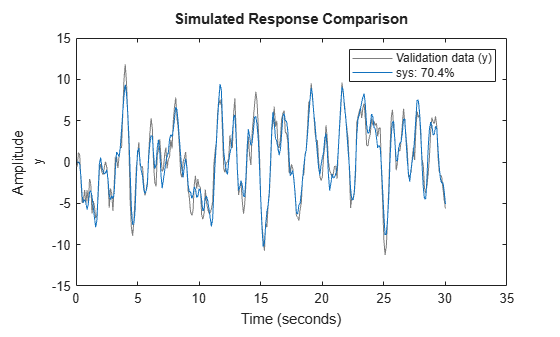The fit now exceeds 70%.

You can view more information about the estimation by exploring the `idproc` property `sys.Report`.

`sys.Report`
```ans = Status: 'Estimated using PROCEST' Method: 'PROCEST' InitialCondition: 'zero' Fit: [1x1 struct] Parameters: [1x1 struct] OptionsUsed: [1x1 idoptions.procest] RandState: [] DataUsed: [1x1 struct] Termination: [1x1 struct] ```

View the estimated gain `Kp`.

`Kp = sys.Kp`
```Kp = 7.6818 ```

Estimate a process model after specifying initial guesses for parameter values and bounding them.

Obtain input/output data.

`data = idfrd(idtf([10 2],[1 1.3 1.2],'iod',0.45),logspace(-2,2,256));`

Specify the parameters of the estimation initialization model.

```type = 'P2UZD'; init_sys = idproc(type); init_sys.Structure.Kp.Value = 1; init_sys.Structure.Tw.Value = 2; init_sys.Structure.Zeta.Value = 0.1; init_sys.Structure.Td.Value = 0; init_sys.Structure.Tz.Value = 1; init_sys.Structure.Kp.Minimum = 0.1; init_sys.Structure.Kp.Maximum = 10; init_sys.Structure.Td.Maximum = 1; init_sys.Structure.Tz.Maximum = 10;```

Specify the estimation options.

```opt = procestOptions('Display','full','InitialCondition','Zero'); opt.SearchMethod = 'lm'; opt.SearchOptions.MaxIterations = 100;```

Estimate the process model.

`sys = procest(data,init_sys,opt);`

Since the `'Display'` option is specified as `'full'`, the estimation progress is displayed in a separate Plant Identification Progress window.

Compare the data to the estimated model.

`compare(data,sys);`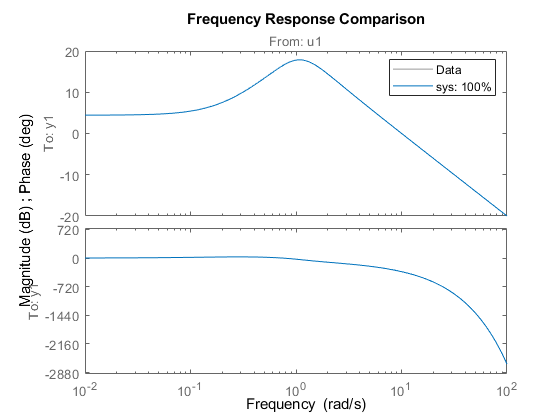```load iddata1 [sys,offset] = procest(z1,'P1DI'); offset```
```offset = 0.0412 ```

`load iddata1ic z1i`

Estimate a first-order plus dead time process model `sys` and return the initial conditions in `ic`. First specify `'estimate'` for `'InitialCondition'` to force the software to estimate `ic`. The default `'auto'` setting uses the `'estimate' method` only when the influence of the initial conditions on the overall model error exceed a threshold. When the initial conditions have a negligible effect on the overall estimation-error minimization process, the `'auto`' setting uses `'zero'`.

```opt = procestOptions('InitialCondition','estimate'); [sys,offset,ic] = procest(z1i,'P1D',opt); ic```
```ic = initialCondition with properties: A: -3.8997 X0: -1.0871 C: 4.5652 Ts: 0 ```

`ic` is an `initialCondition` object that encapsulates the free response of `sys`, in state-space form, to the initial state vector in `X0`. You can incorporate `ic` when you simulate `sys` with the `z1i` input signal and compare the response with the `z1i` output signal.

Obtain input/output data.

```load iddata1 z1 load iddata2 z2 data = [z1 z2(1:300)];```

`data` is a data set with 2 inputs and 2 outputs. The first input affects only the first output. Similarly, the second input affects only the second output.

In the estimated process model, the cross terms, which model the effect of the first input on the second output and vice versa, should be negligible. If the estimation process instead assigns higher orders to the cross dynamics, the degrees of estimation uncertainty for those terms should be high.

Estimate the process model.

```type = 'P2UZ'; sys = procest(data,type);```

The `type` variable denotes a model with complex-conjugate pair of poles, a zero, and a delay.

To evaluate the uncertainties, plot the frequency response.

```w = linspace(0,20*pi,100); h = bodeplot(sys,w); showConfidence(h);```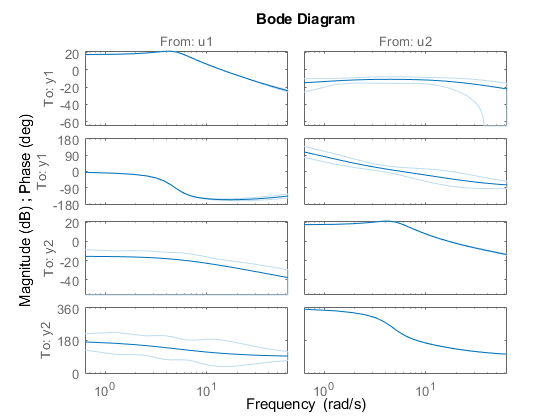The responses from the cross pairs show larger uncertainty, indicating that using a single `type` for each input/output pair results in too much energy in the cross pairs.

Use regularization to estimate parameters of an overparameterized process model.

`load iddata1 z1;`

Construct an initial system `sysi` by specifying parameter values for a model that includes three poles, one zero, and underdamped modes. Assume that gain `Kp` is known with a higher degree of confidence than the other model parameters.

`sysi = idproc('P3UZ','Kp',7.5,'Tw',0.25,'Zeta',0.3,'Tp3',20,'Tz',0.02);`

Estimate an unregularized process model `sys1 `using `sysi` to initialize the estimation model.

`sys1 = procest(z1,sysi);`

Estimate a regularized process model `sys2` from `sysi`. Because `K` has a higher level of confidence, set the regularization constant `R` higher than for the other model parameters. This setting causes the estimation process to place more emphasis on maintaining the initial value of `K`.

```opt = procestOptions; opt.Regularization.Nominal = 'model'; opt.Regularization.R = [100;1;1;1;1]; opt.Regularization.Lambda = 0.1; sys2 = procest(z1,sysi,opt);```

Compare the model outputs with data.

`compare(z1,sys1,sys2);`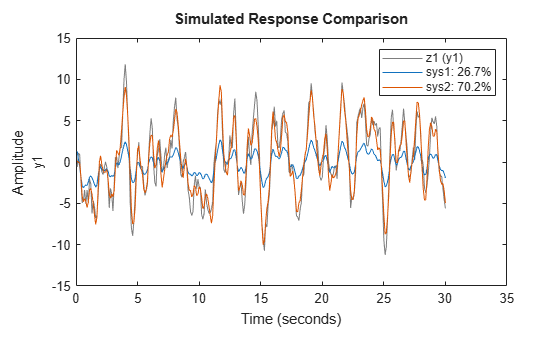Regularization helps steer the estimation process towards the correct parameter values, as the better fit for `sys2` shows.

Compare the estimated gain values for `sys1` and `sys2`.

`g1 = sys1.Kp`
```g1 = -0.2320 ```
`g2 = sys2.Kp`
```g2 = 6.6236 ```

The `Kp` value for the regularized system is much closer to the initial value than for the unregularized system.

Obtain the measured input-output data.

```load iddemo_heatexchanger_data; data = iddata(pt,ct,Ts); data.InputName = '\Delta CTemp'; data.InputUnit = 'C'; data.OutputName = '\Delta PTemp'; data.OutputUnit = 'C'; data.TimeUnit = 'minutes';```

Estimate a first-order plus dead time process model.

```type = 'P1D'; sysP1D = procest(data,type);```

Compare the model with the data.

`compare(data,sysP1D)`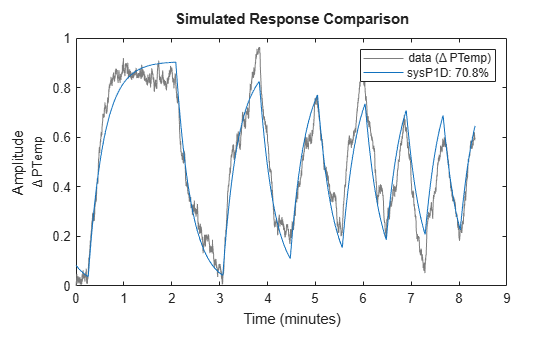Plot the model residuals.

```figure resid(data,sysP1D);```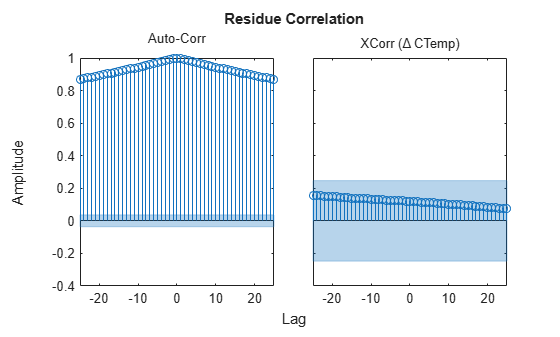The figure shows that the residuals are correlated. To account for that, add a first order ARMA disturbance component to the process model.

```opt = procestOptions('DisturbanceModel','ARMA1'); sysP1D_noise = procest(data,'p1d',opt);```

Compare the models.

`compare(data,sysP1D,sysP1D_noise)`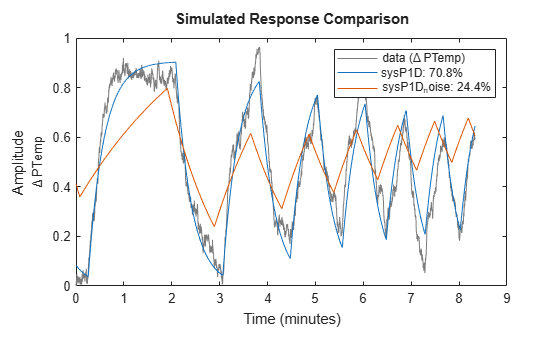Plot the model residuals.

```figure resid(data,sysP1D_noise);```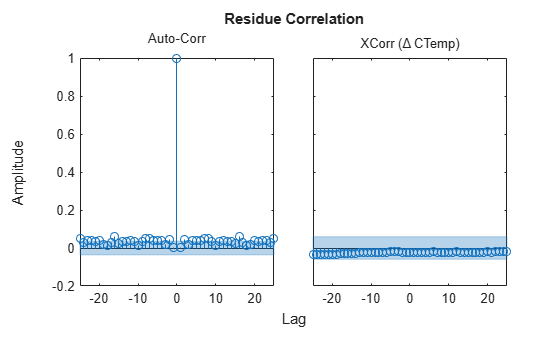The residues of `sysP1D_noise` are uncorrelated.

## Input Arguments

collapse all

Estimation data, specified as a uniformly sampled `timetable` that contains both input and output signal variables or, for multiexperiment data, a cell array of timetables.

#### Use Entire Timetable

If you want to use all the input and output variables in `tt`, and the variables are organized so that the set of input variables is followed by the set of output variables, then:

• For SISO systems, specify `tt` as an Ns-by-2 `timetable`, where Ns is the number of samples and the two `timetable` variables represent the measured input signal and output signal respectively.

• For MIMO systems, specify `tt` as an Ns-by-(Nu+Ny) `timetable`, where Nu is the number of inputs and Ny is the number of outputs. The first Nu variables must contain the input signals and the remaining Ny variables must contain the output signals.

When you are estimating state space or transfer function models, you must also explicitly specify the input and output channels, as the following section describes.

• For multiexperiment data, specify data as an Ne-by-1 cell array of timetables, where Ne is the number of experiments. The sample times of all the experiments must match.

#### Use Selected Variables from Timetable

If you want to use a subset of variables from the `timetable`, or if the input and output variables are intermixed, use the `'InputName'` and `'OutputName'` name-value arguments to specify which variables to use.

For example, suppose that `tt` contains six variables: `"u1"`, `"u2"`, `"u3"`, and `"y1"`, `"y2"`, `"y3"`. For estimation, you want to use the variables `"u1"` and `"u2"` as the inputs and the variables `"y1"` and `"y3"` as the outputs. Use the following command to perform the estimation:

```sys = procest(tt,__,'InputName',["u1" "u2"],'OutputName',["y1" "y3"])```

For more information about working with estimation data types, see Data Domains and Data Types in System Identification Toolbox.

Estimation data object, specified as an `iddata` object, an `frd` object, or an `idfrd` object that contains uniformly sampled input and output values. By default, the software sets the sample time of the model to the sample time of the estimation data.

For multiexperiment data, the sample times and intersample behavior of all the experiments must match.

For time-domain estimation, `data` must be an `iddata` object containing the input and output signal values.

For frequency-domain estimation, `data` can be one of the following:

• Recorded frequency response data (`frd` (Control System Toolbox) or `idfrd`)

• `iddata` object with properties specified as follows:

• `InputData` — Fourier transform of the input signal

• `OutputData` — Fourier transform of the output signal

• `Domain``'Frequency'`

#### Limitations

You cannot estimate continuous-time models using discrete-time frequency-domain data.

Process model structure, specified for SISO models as a string or character vector that represents an acronym for the model structure, such as `'P1D'` or `'P2DZ'`. The acronym starts with `P` and can contain any combination of the other following components:

• `P` — Poles. All `'Type'` acronyms start with `P`, because all process modes must have at least one pole.

• `0`, `1`, `2`, or `3` — Number of time constants (poles) to be modeled. This number does not include possible integrations (poles in the origin).

• `I` — Integration is enforced (self-regulating process).

• `D` — Time delay (dead time).

• `Z` — Extra numerator term, a zero.

• `U` — Underdamped modes (complex-valued poles) permitted. If `U` is not included in `type`, all poles must be real. The number of poles must be 2 or 3.

For MIMO models, specify `type` as an Ny-by-Nu cell array of character vectors or string array, with one entry for each input-output pair. Here Ny is the number of inputs and Nu is the number of outputs.

For information regarding how `type` affects the structure of a process model, see `idproc`.

Process model that configures initial parameterization of `sys`, specified as an `idproc` object. You obtain `init_sys` by either performing an estimation using measured data or by direct construction using `idproc`. The software uses the parameters and constraints defined in `init_sys` as the initial guess for estimating `sys`.

Use the `Structure` property of `init_sys` to configure initial guesses and constraints for Kp, Tp1, Tp2, Tp3, Tw, ζ, Td, and Tz. For example:

• To specify an initial guess for the Tp1 parameter of `init_sys`, set `init_sys.Structure.Tp1.Value` as the initial guess.

• To specify constraints for the Tp2 parameter of `init_sys`:

• Set `init_sys.Structure.Tp2.Minimum` to the minimum Tp2 value.

• Set `init_sys.Structure.Tp2.Maximum` to the maximum Tp2 value.

• Set `init_sys.Structure.Tp2.Free` to indicate if Tp2 is a free parameter for estimation.

If you do not specify `opt`, and `init_sys` was obtained by estimation rather than construction, then the software uses estimation options from `init_sys.Report.OptionsUsed`

Estimation options, specified as an `procestOptions` option set. The estimation options include:

• Estimation objective

• Handling on initial conditions and disturbance component

• Numerical search method to be used in estimation

• Intersample behavior

### Name-Value Arguments

Specify optional pairs of arguments as `Name1=Value1,...,NameN=ValueN`, where `Name` is the argument name and `Value` is the corresponding value. Name-value arguments must appear after other arguments, but the order of the pairs does not matter.

Before R2021a, use commas to separate each name and value, and enclose `Name` in quotes.

Example: `sys = procest(tt,'P1D,'InputDelay',2)`

Input channel names, specified as a string, character vector, string array, or cell array of character vectors.

If you are using a timetable for the data source, the names in `InputName` must be a subset of the timetable variables.

Example: `sys = procest(tt,__,'InputName',["u1" "u2"])` selects the variables `u1` and `u2` as the input channels from the timetable `tt` to use for the estimation.

Output channel names, specified as a string, character vector, string array, or cell array of character vectors.

If you are using a timetable for the data source, the names in `OutputName` must be a subset of the timetable variables.

Example: `sys = procest(tt,__,'OutputName',["y1" "y3"])` selects the variables `y1` and `y3` as the output channels from the timetable `tt` to use for the estimation.

Input delays, specified as a numeric vector specifying a time delay for each input channel. Specify input delays in the time unit stored in the `TimeUnit` property.

For a system with Nu inputs, set `InputDelay` to an Nu-by-1 vector. Each entry of this vector is a numerical value that represents the input delay for the corresponding input channel. You can also set `InputDelay` to a scalar value to apply the same delay to all channels.

The software treats `InputDelay` as a fixed delay that is separate from any transport delay that the `Td` property of the model introduces.

## Output Arguments

collapse all

Identified process model, returned as an `idproc` model of a structure defined by `type`.

Information about the estimation results and options used is stored in the model's `Report` property. `Report` has the following fields:

Report FieldDescription
`Status`

Summary of the model status, which indicates whether the model was created by construction or obtained by estimation.

`Method`

Estimation command used.

`InitialCondition`

Handling of initial conditions during model estimation, returned as one of the following values:

• `'zero'` — The initial conditions were set to zero.

• `'estimate'` — The initial conditions were treated as independent estimation parameters.

• `'backcast'` — The initial conditions were estimated using the best least squares fit.

This field is especially useful to view how the initial conditions were handled when the `InitialCondition` option in the estimation option set is `'auto'`.

`Fit`

Quantitative assessment of the estimation, returned as a structure. See Loss Function and Model Quality Metrics for more information on these quality metrics. The structure has the following fields:

FieldDescription
`FitPercent`

Normalized root mean squared error (NRMSE) measure of how well the response of the model fits the estimation data, expressed as the percentage fitpercent = 100(1-NRMSE).

`LossFcn`

Value of the loss function when the estimation completes.

`MSE`

Mean squared error (MSE) measure of how well the response of the model fits the estimation data.

`FPE`

Final prediction error for the model.

`AIC`

Raw Akaike Information Criteria (AIC) measure of model quality.

`AICc`

Small-sample-size corrected AIC.

`nAIC`

Normalized AIC.

`BIC`

Bayesian Information Criteria (BIC).

`Parameters`

Estimated values of model parameters.

`OptionsUsed`

Option set used for estimation. If no custom options were configured, this is a set of default options. See `procestOptions` for more information.

`RandState`

State of the random number stream at the start of estimation. Empty, `[]`, if randomization was not used during estimation. For more information, see `rng`.

`DataUsed`

Attributes of the data used for estimation. Structure with the following fields:

FieldDescription
`Name`

Name of the data set.

`Type`

Data type. For `idnlarx` models, this is set to `'Time domain data'`.

`Length`

Number of data samples.

`Ts`

Sample time. This is equivalent to `Data.Ts`.

`InterSample`

Input intersample behavior. One of the following values:

• `'zoh'` — Zero-order hold maintains a piecewise-constant input signal between samples.

• `'foh'` — First-order hold maintains a piecewise-linear input signal between samples.

• `'bl'` — Band-limited behavior specifies that the continuous-time input signal has zero power above the Nyquist frequency.

The value of `Intersample` has no effect on estimation results for discrete-time models.

`InputOffset`

Empty, `[]`, for nonlinear estimation methods.

`OutputOffset`

Empty, `[]`, for nonlinear estimation methods.

`Termination`

Termination conditions for the iterative search used for prediction error minimization, returned as a structure with the following fields:

FieldDescription
`WhyStop`

Reason for terminating the numerical search.

`Iterations`

Number of search iterations performed by the estimation algorithm.

`FirstOrderOptimality`

$\infty$-norm of the gradient search vector when the search algorithm terminates.

`FcnCount`

Number of times the objective function was called.

`UpdateNorm`

Norm of the gradient search vector in the last iteration. Omitted when the search method is `'lsqnonlin'` or `'fmincon'`.

`LastImprovement`

Criterion improvement in the last iteration, expressed as a percentage. Omitted when the search method is `'lsqnonlin'` or `'fmincon'`.

`Algorithm`

Algorithm used by `'lsqnonlin'` or `'fmincon'` search method. Omitted when other search methods are used.

For estimation methods that do not require numerical search optimization, the `Termination` field is omitted.

For more information on using `Report`, see Estimation Report.

Estimated value of input offset, returned as a vector. When `data` has multiple experiments, `offset` is a matrix where each column corresponds to an experiment.

Estimated initial conditions, returned as an `initialCondition` object or an object array of `initialCondition` values.

• For a single-experiment data set, `ic` represents, in state-space form, the free response of the process model (A and C matrices) to the estimated initial states (x0).

• For a multiple-experiment data set with Ne experiments, `ic` is an object array of length Ne that contains one set of `initialCondition` values for each experiment.

If `procest` returns `ic` values of `0` and the you know that you have non-zero initial conditions, set the `'InitialCondition'` option in `procestOptions` to `'estimate'` and pass the updated option set to `procest`. For example:

```opt = procestOptions('InitialCondition','estimate') [sys,offset,ic] = procest(data,type,opt)```
The default `'auto'` setting of `'InitialCondition'` uses the `'zero'` method when the initial conditions have a negligible effect on the overall estimation-error minimization process. Specifying `'estimate'` ensures that the software estimates values for `ic`.

For more information, see `initialCondition`. For an example of using this argument, see Obtain Initial Conditions.

## Version History

Introduced in R2012a

expand all